We think you are located in United States. Is this correct?

# 9.4 Titrations

## 9.4 Titrations (ESCPJ)

### What are titrations? (ESCPK)

The neutralisation reaction between an acid and a base can be very useful. If an acidic solution of known concentration (a standard solution) is added to a basic (alkaline) solution of unknown concentration until the solution is exactly neutralised (i.e. there is only salt and water), it is possible to calculate the exact concentration of the unknown solution. It is possible to do this because, at the exact point where the solution is neutralised, stoichiometrically equivalent mole amounts of acid and base have reacted with each other.

Titration

The method used to determine the concentration of a known substance using another, standard, solution.

In a titration: a known volume of a standard solution (A) is added to a known volume of a solution with unknown concentration (B). The concentration of B can then be determined.

Acids and bases are commonly used in titrations, and the point of neutralisation is called the end-point of the reaction. If you have an indicator that changes colour in the range of the end-point pH then you will be able to see when the end-point has occurred. Another name for a titration is volumetric analysis.

### Titration calculations (ESCPM)

So how exactly can a titration be carried out to determine an unknown concentration? Look at the following steps to help you to understand the process.

1. A carefully measured volume of the solution with unknown concentration is put into a conical flask.

2. A few drops of a suitable indicator is added to this solution (bromothymol blue and phenolphthalein are common indicators, refer to Table 9.9).

3. The conical flask is placed on a white tile or piece of paper (to make colour changes easier to see).

4. A volume of the standard solution (known concentration) is put into a burette (a measuring device) and is slowly added to the solution in the flask, drop by drop.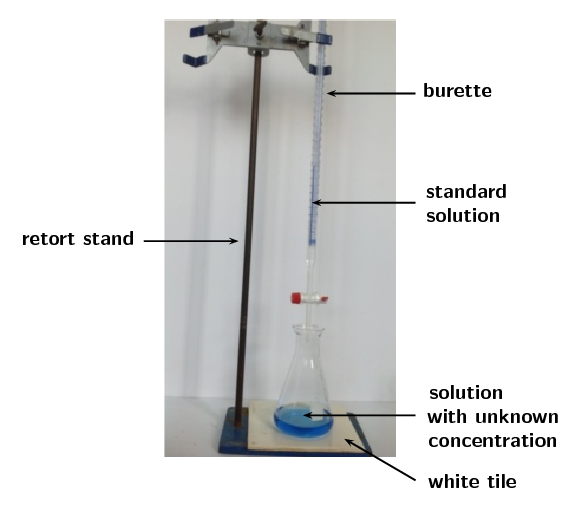It is better to have the acid in the burette as many bases will clog the stopcock and damage the burette. It is much easier to clean a burette that has contained acid only. It is important to make sure the burette is perpendicular to the desk and clamped securely.

When filling the burette the stopcock should be closed and the funnel should be lifted slightly to allow air to escape, otherwise it will bubble through the liquid in the funnel and splash acid. Once the burette is filled a beaker should be placed underneath and a few centimeters of the solution should be drained through the stopcock. This ensures that the entire burette is full. There should be no air bubbles, as a bubble bursting can affect the volume measurement and disrupt the accuracy of the experiment.

5. At some point, adding one more drop will change the colour of the unknown solution to the colour of the end-point of the reaction. Remember the colour changes from Figure 9.3.

6. Record the volume of standard solution that has been added up to this point.

7. Use the information you have gathered to calculate the exact concentration of the unknown solution. Worked examples are given to walk you through this step.

8. Note that adding more solution once the end-point has been reached will result in a colour change from the end-point colour to that of the acid (if the solution in the conical flask is a base) or of the base (if the solution in the conical flask is an acid).

When you are busy with these calculations, you will need to remember the following:

$$\text{1}$$ $$\text{dm^{3}}$$ = $$\text{1}$$ $$\text{ℓ}$$ = $$\text{1 000}$$ $$\text{mL}$$ = $$\text{1 000}$$ $$\text{cm^{3}}$$, therefore dividing $$\text{cm^{3}}$$ by $$\text{1 000}$$ will give you an answer in $$\text{dm^{3}}$$.

Some other terms and equations which will be useful to remember are shown below:

• concentration of a solution is measured in $$\text{mol·dm^{-3}}$$

• moles (mol) $$=$$ concentration ($$\text{mol·dm^{-3}}$$) $$\times$$ volume ($$\text{dm^{3}}$$)

• \begin{align*} \text{concentration } = \frac{\text{moles}}{\text{volume}} \\ c (\text{mol·dm$^{-3}$}) = \frac{n (\text{mol})}{V (\text{dm$^{3}$})} \end{align*}
• remember to make sure all the units are correct in your calculations

## Worked example 13: Titration calculations

Given the equation:

$$\text{NaOH}(\text{aq}) + \text{HCl}(\text{aq})$$ $$\to$$ $$\text{NaCl}(\text{aq}) + \text{H}_{2}\text{O}(\text{ℓ})$$

$$\text{25}$$ $$\text{cm^{3}}$$ of sodium hydroxide solution was pipetted into a conical flask and titrated with $$\text{0,2}$$ $$\text{mol·dm^{-3}}$$ hydrochloric acid. Using a suitable indicator, it was found that $$\text{15}$$ $$\text{cm^{3}}$$ of acid was needed to neutralise the base. Calculate the concentration of the sodium hydroxide.

### Make sure that the equation is balanced

There are equal numbers of each type of atom on each side of the equation, so the equation is balanced.

### Write down all the information you know about the reaction, converting to the correct units

NaOH: $$V = \text{25}$$ $$\text{cm^{3}}$$ $$\times \dfrac{\text{0,001} {\text{ dm}}^{3}}{\text{1} {\text{ cm}}^{3}}$$ = $$\text{0,025}$$ $$\text{dm^{3}}$$

HCl: $$V = \text{15}$$ $$\text{cm^{3}}$$ $$\times \dfrac{\text{0,001} {\text{ dm}}^{3}}{\text{1} {\text{ cm}}^{3}}$$ = $$\text{0,015}$$ $$\text{dm^{3}}$$

$$\phantom{\rule{25pt}{0ex}}$$$$c = \text{0,2}$$ $$\text{mol·dm^{-3}}$$

### Calculate the number of moles of HCl that are added

$$c = \dfrac{n}{V}$$

Therefore, $$n(\text{HCl})$$ = $$c \times V$$

n(HCl) = $$\text{0,2}$$ $$\text{mol·dm^{-3}}$$ $$\times$$ $$\text{0,015}$$ $$\text{dm^{3}}$$ = $$\text{0,003}$$ $$\text{mol}$$

$$\text{0,003}$$ $$\text{moles}$$ of $$\text{HCl}$$ are required to neutralise the base.

### Calculate the number of moles of sodium hydroxide in the reaction

Look at the equation for the reaction: the molar ratio of $$\text{HCl}$$:$$\text{NaOH}$$ is $$\text{1}$$:$$\text{1}$$.

So for every mole of $$\text{HCl}$$, there is one mole of $$\text{NaOH}$$ that is involved in the reaction. Therefore, if $$\text{0,003}$$ $$\text{mol}$$ $$\text{HCl}$$ is required to neutralise the solution, $$\text{0,003}$$ $$\text{mol}$$ $$\text{NaOH}$$ must have been present in the sample of the unknown solution.

### Calculate the concentration of the sodium hydroxide solution

$${c\text{(NaOH)}} = \dfrac{n}{V} = \dfrac{\text{0,003}\text{ mol}}{\text{0,025}\text{ dm^{3}}} = \text{0,12}\text{ mol·dm^{-3}}$$

The concentration of the $$\text{NaOH}$$ solution is $$\text{0,12}$$ $$\text{mol·dm^{-3}}$$

## Worked example 14: Titration calculations

$$\text{10}$$ $$\text{g}$$ of solid sodium hydroxide is dissolved in $$\text{500}$$ $$\text{cm^{3}}$$ water. Using titration, it was found that $$\text{20}$$ $$\text{cm^{3}}$$ of this solution was able to completely neutralise $$\text{10}$$ $$\text{cm^{3}}$$ of a sulfuric acid solution. Calculate the concentration of the sulfuric acid.

### Write a balanced equation for the titration reaction

The reactants are sodium hydroxide ($$\text{NaOH}$$) and sulfuric acid ($$\text{H}_{2}\text{SO}_{4}$$). The base has a hydroxide anion ($$\text{OH}^{-}$$), therefore the products will be a salt and water.

The cation for the salt ($$\text{Na}^{+}$$) will come from the base. The anion for the salt ($$\text{SO}_{4}^{2-}$$) will come from the acid. There must be $$\text{2}$$ $$\text{Na}^{+}$$ cations for every one $$\text{SO}_{4}^{2-}$$ and the salt will be $$\text{Na}_{2}\text{SO}_{4}$$.

$$\text{H}_{2}\text{SO}_{4}(\text{aq}) + \text{NaOH}(\text{aq})$$ $$\to$$ $$\text{Na}_{2}\text{SO}_{4}(\text{aq}) + \text{H}_{2}\text{O}(\text{ℓ})$$

To balance the equation we need to multipy the number of sodium hydroxide molecules and the water molecules by two.

$$\text{H}_{2}\text{SO}_{4}(\text{aq}) + 2\text{NaOH}(\text{aq})$$ $$\to$$ $$\text{Na}_{2}\text{SO}_{4}(\text{aq}) + 2\text{H}_{2}\text{O}(\text{ℓ})$$

### Calculate the concentration of the sodium hydroxide solution

The total volume that the $$\text{10}$$ $$\text{g}$$ was dissolved in must be used to calculate the concentration.

$$V = \text{500}$$ $$\text{cm^{3}}$$ $$\times \dfrac{\text{0,001} {\text{ dm}}^{3}}{\text{1} {\text{ cm}}^{3}}$$ = $$\text{0,5}$$ $$\text{dm^{3}}$$

$$M(\text{NaOH}) = \text{23,0}$$ + $$\text{16,0}$$ + $$\text{1,01}$$ = $$\text{40,01}$$ $$\text{g.mol^{-1}}$$

$$n(\text{NaOH}) = \dfrac{\text{m}}{\text{M}} = \dfrac{\text{10}\text{ g}}{\text{40,01}\text{ g.mol^{-1}}} = \text{0,25}\text{ mol}$$

$$c(\text{NaOH}) = \dfrac{\text{n}}{\text{V}} = \dfrac{\text{0,25}\text{ mol}}{\text{0,5}\text{ dm^{3}}} = \text{0,50}\text{ mol·dm^{-3}}$$

### Calculate the number of moles of sodium hydroxide that were used in the neutralisation reaction

Remember that only $$\text{20}$$ $$\text{cm^{3}}$$ of the sodium hydroxide solution is used:

$$V = \text{20}$$ $$\text{cm^{3}}$$ $$\times \dfrac{\text{0,001} {\text{ dm}}^{3}}{\text{1} {\text{ cm}}^{3}}$$ = $$\text{0,02}$$ $$\text{dm^{3}}$$

$$c = \dfrac{n}{V}$$, therefore $$n = {c} \times {\text{V}}$$

$$n = \text{0,50}\text{ mol·dm^{-3}} \times \text{0,02}\text{ dm^{3}} = \text{0,01}\text{ mol}$$

### Calculate the number of moles of sulfuric acid that were neutralised

According to the balanced chemical equation, the mole ratio of $$\text{NaOH}$$ to $$\text{H}_{2}\text{SO}_{4}$$ is $$\text{2}$$:$$\text{1}$$. There are $$\text{2}$$ moles of $$\text{NaOH}$$ for every $$\text{1}$$ mole of $$\text{H}_{2}\text{SO}_{4}$$.

$$n(\text{H}_{2}\text{SO}_{4}) = \dfrac{\text{0,01}\text{ mol}}{\text{2}}$$ = $$\text{0,005}$$ $$\text{mol}$$.

### Calculate the concentration of the sulfuric acid solution

Remember that $$\text{10}$$ $$\text{cm^{3}}$$ of the sulfuric acid solution is neutralised.

$$V(\text{H}_{2}\text{SO}_{4}) = \text{10}$$ $$\text{cm^{3}}$$ $$\times \dfrac{\text{0,001} {\text{ dm}}^{3}}{\text{1} {\text{ cm}}^{3}}$$ = $$\text{0,01}$$ $$\text{dm^{3}}$$

$$C = \dfrac{n}{V} = \dfrac{\text{0,005}\text{ mol}}{\text{0,01}\text{ dm^{3}}} = \text{0,5}\text{ mol·dm^{-3}}$$

The formal assessment requires that the learners prepare a standard solution of sodium hydroxide. It is important that the learners understand that in order for the concentration of a standard solution to be accurately known:

• The mass must be precisely measured.

• The beakers must be rinsed with water (and the rinsings added to the volumetric flask) so that no residue of the sodium hydroxide is left behind.

• The sides of the volumetric flask must be washed with water for the same reason.

• It is important to have their eye level with the mark on the volumetric flask when filling to that mark so that the volume is precise.

Learners are working with a strong base in this reaction. Concentrated, strong bases can cause serious burns. Please remind the learners to be careful and wear the appropriate safety equipment when handling all chemicals, especially concentrated bases. The safety equipment includes gloves, safety glasses, and protective clothing.

temp text

## Preparation of a standard solution

### Aim

To prepare a standard solution of sodium hydroxide.

### Apparatus and materials

• one $$\text{250}$$ $$\text{cm^{3}}$$ volumetric flask, a beaker, a funnel, a plastic dropper

• A weighing scale, a spatula, a clean piece of paper

• solid sodium hydroxide

### Method

Concentrated bases can cause serious burns. We suggest using gloves and safety glasses whenever you work with a base. Handle all chemicals with care.

1. Place the paper on the scale and tare (zero) the scale.

2. Weigh $$\text{5,00}$$ $$\text{g}$$ of solid sodium hydroxide onto the paper.

3. Transfer the sodium hydroxide to the beaker and dissolve it in a minimal amount of water.

4. Place the funnel in the mouth of the volumetric flask and use it to transfer the solution from the beaker to the flask, be careful not to spill.

5. Pour another $$\text{5}$$ $$\text{cm^{3}}$$ of water into the beaker, swirl gently, and pour this into the flask through the funnel as well.

6. Repeat step $$\text{5}$$ another two times.

7. Pour $$\text{5}$$ $$\text{cm^{3}}$$ of water through the funnel into the flask.

8. Use the plastic dropper to pour water down the inside of the volumetric flask.

9. Fill the volumetric flask to the mark with water. Use the plastic dropper to fill the last few centimeters. Remember to lower yourself so that you are looking directly at the mark when adding the final drops.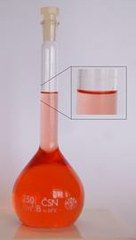10. Cap the flask and shake well. You now have a standard solution.

### Questions

• What is the concentration of this standard solution?

• What is the purpose of rinsing the beaker and funnel with water?

• What is the purpose of rinsing the inside of the volumetric flask with water?

• Why do you need to make sure your eyes are level with the mark when adding the final few drops of water?

A less accurate alternative to the titration of acetic acid with sodium hydroxide experiment is provided here (if titration equipment is not available).

## Titrations

### Aim

To determine the concentration of acetic acid (ethanoic acid) in a sample of vinegar.

### Apparatus and materials

• 4 conical flasks, plastic droppers, two measuring cylinders

• a white piece of paper or a white tile

• the standard $$\text{NaOH}$$ solution prepared in the previous experiment, white vinegar, phenolphthalein

### Method

Concentrated acids and bases can cause serious burns. We suggest using gloves and safety glasses whenever you work with an acid or a base. Handle all chemicals with care.

1. Label a conical flask 1 and add $$\text{20}$$ $$\text{cm^{3}}$$ of the sodium hydroxide solution to the conical flask using a measuring cylinder.

2. Add a few drops of the phenolphthalein indicator to conical flask 1.

3. Measure $$\text{10}$$ $$\text{cm^{3}}$$ vinegar in the second measuring cylinder.

4. Pour the $$\text{10}$$ $$\text{cm^{3}}$$ vinegar into conical flask 1. Swirl the flask.

5. If there is no colour change measure $$\text{5}$$ $$\text{cm^{3}}$$ vinegar in the measuring cylinder and add this to conical flask 1. Swirl the flask.

6. Repeat step $$\text{5}$$ until the colour change remains after swirling. Calculate the volume of vinegar added. This is your rough titration.

7. Repeat steps $$\text{1}$$ and $$\text{2}$$ with a second conical flask (label it 2).

8. Add a volume of vinegar no more than $$\text{5}$$ $$\text{cm^{3}}$$ less than your rough titration volume to conical flask 2. This is your initial volume added.

9. Measure $$\text{10}$$ $$\text{cm^{3}}$$ of vinegar in the second measuring cylinder.

10. Use a dropper to transfer vinegar from the measuring cylinder to the conical flask. Swirl between each drop and be careful not to spill. If you get vinegar on the side of the conical flask, rinse with water.

11. When there is a permanent colour change after swirling, the end-point has been reached. Determine the volume added:

Total volume added = initial volume added + ($$\text{10}$$ $$\text{cm^{3}}$$ - final volume in measuring cylinder)

12. Repeat steps $$\text{7}$$ - $$\text{11}$$, labelling the conical flasks 3 and 4.

### Continue with the rest of the experiment as laid out in the learners textbook

Although this method will not be as accurate, it will give the learners an idea of what a titration is like.

Different concentrations of the standard solution may be used, and the calculations will have to be adjusted in that case.

It is possible to put the sodium hydroxide in the burette and the vinegar in the conical flask. This is likely to clog the burette though, so if you do this make sure to wash your burette thoroughly after use to avoid the stopcock being damaged. It may be necessary to drain a few $$\text{cm^{3}}$$ of the vinegar to remove all air bubbles from the burette. In this case add more vinegar to the burette after removing the air bubbles so that the top of the vinegar is between the $$\text{0}$$ $$\text{cm^{3}}$$ and $$\text{1}$$ $$\text{cm^{3}}$$ marks.

Learners are working with a strong base in this reaction. Concentrated, strong bases can cause serious burns. Please remind the learners to be careful and wear the appropriate safety equipment when handling all chemicals, especially strong, concentrated bases. The safety equipment includes gloves, safety glasses, and protective clothing.

## Titrations

### Aim

To determine the concentration of acetic acid (ethanoic acid) in a sample of vinegar.

### Apparatus and materials

• 4 conical flasks, one pipette, one burette, one small funnel, one beaker

• a retort stand, a white piece of paper or a white tile

• the standard $$\text{NaOH}$$ solution prepared in the previous experiment, white vinegar, phenolphthalein

### Method

Concentrated acids and bases can cause serious burns. We suggest using gloves and safety glasses whenever you work with an acid or a base. Handle all chemicals with care.

1. Clamp the burette to the retort stand and place the small funnel on top.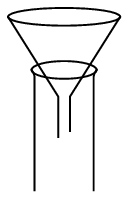2. Making sure the burette is closed, carefully add the vinegar to the burette (lift the funnel slightly while doing this) until the burette is full.

3. Place a beaker below the burette and carefully let some of the vinegar run into it. This will ensure that there are no air bubbles in the burette. The top of the vinegar should now be between the $$\text{0}$$ and $$\text{1}$$ $$\text{cm^{3}}$$ marks. Record the value to the second decimal place.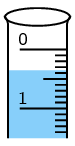4. Use your pipette to measure $$\text{20}$$ $$\text{cm^{3}}$$ of the sodium hydroxide standard solution into a conical flask.

5. Add $$\text{3}$$ - $$\text{4}$$ drops of phenolphthalein indicator to the conical flask. What colour is the solution?

6. Do a rough titration experiment by adding the vinegar to the conical flask quickly, and constantly swirling the conical flask. Stop as soon as the colour of the solution changes, and remains changed after swirling. Make a note of the reading on the burette at this point and determine the volume added:

(V(final) - V(initial)).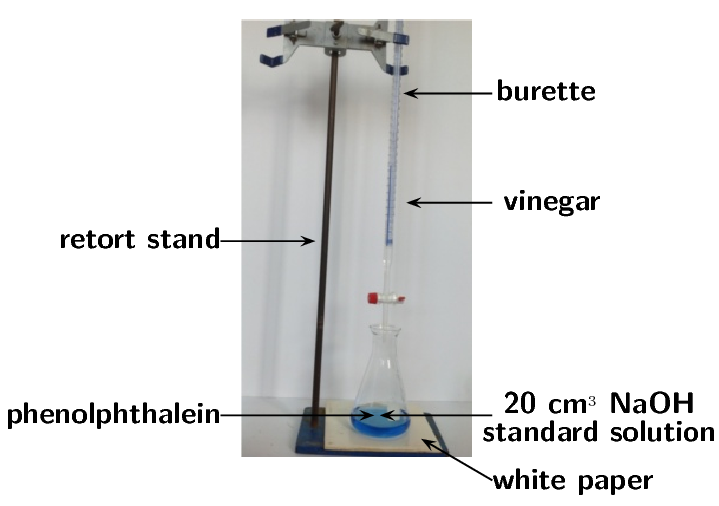If the colour change does not remain when the flask is swirled, add more vinegar until the colour remains.

7. Repeat steps $$\text{2}$$ - $$\text{5}$$ with a second conical flask.

8. Quickly add vinegar to the conical flask until you have added $$\text{2}$$ $$\text{cm^{3}}$$ less than your rough titration volume. There should be no lasting colour change at this point.

9. Drop by drop (slowly and carefully), add vinegar. Swirl between each drop and, if necessary, rinse the sides of the flask with water. When the solution changes colour and remains that new colour, make a note of the volume on the burette.

Remember that the volume titrated is: (final volume reading) - (the initial volume reading).

10. For accuracy you should repeat steps $$\text{7}$$ - $$\text{9}$$ until you have three readings with a difference of no more than $$\text{0,1}$$ $$\text{cm^{3}}$$.

### Observations and questions

• What colour was the sodium hydroxide solution when the phenolphthalein was added?

• What was the colour when enough acid was added?

Fill in the details of this experiment on a table like the one below:

 Titration Initial volume ($$\text{cm^{3}}$$) Final volume ($$\text{cm^{3}}$$) Volume ($$\text{cm^{3}}$$) Rough 1 2 3
• From the table determine the average titration volume for this experiment and use that value in the rest of your calculations.

• Using the previous worked examples, determine the concentration of acetic acid in the sample of vinegar. Remember that you have the following information:

• the volume of vinegar

• the volume of the sodium hydroxide solution

• the concentration of the sodium hydroxide solution

• The balanced chemical equation for this reaction is:

$$\text{CH}_{3}\text{COOH}(\text{aq}) + \text{NaOH}(\text{aq})$$ $$\to$$ $$\text{CH}_{3}\text{COONa}(\text{aq}) + \text{H}_{2}\text{O}(\text{ℓ})$$

A less accurate alternative to the titration of sodium hydroxide with oxalic acid experiment is provided here (if titration equipment is not available).

## Titrations

### Aim

To determine the concentration of a sodium hydroxide solution of unknown concentration.

### Apparatus and materials

• one $$\text{250}$$ $$\text{cm^{3}}$$ volumetric flask, 4 conical flasks, two plastic droppers, two measuring cylinders, one beaker

• a weighing scale, a spatula, a clean piece of paper, a white sheet of paper or a white tile

• solid sodium hydroxide ($$\text{NaOH}$$), solid oxalic acid ($$\text{H}_{2}\text{C}_{2}\text{O}_{ 4}$$), phenolphthalein

### Method

Concentrated acids and bases can cause serious burns. We suggest using gloves and safety glasses whenever you work with an acid or a base. Handle all chemicals with care.

1. Prepare a standard solution using $$\text{11,00}$$ $$\text{g}$$ of oxalic acid in the $$\text{250}$$ $$\text{cm^{3}}$$ volumetric flask (remember to follow the method from the formal preparation of a standard solution experiment).

2. Label one of the beakers $$\text{NaOH}$$. Use a measuring cylinder to measure approximately $$\text{100}$$ $$\text{cm^{3}}$$ of water into the beaker. Add approximately $$\text{4}$$ $$\text{g}$$ of $$\text{NaOH}$$ to the beaker and stir.

3. Label a conical flask 1 and transfer $$\text{20}$$ $$\text{cm^{3}}$$ of the sodium hydroxide to the conical flask.

4. Add a few drops of the phenolphthalein indicator to conical flask 1.

5. Measure $$\text{10}$$ $$\text{cm^{3}}$$ of the oxalic acid solution in the second measuring cylinder.

6. Pour the $$\text{10}$$ $$\text{cm^{3}}$$ oxalic acid solution into conical flask 1. Swirl the flask.

7. If there is no colour change measure $$\text{5}$$ $$\text{cm^{3}}$$ oxalic acid in the measuring cylinder and add this to conical flask 1. Swirl the flask.

8. Repeat step $$\text{7}$$ until the colour change remains after swirling. Calculate the volume of oxalic acid solution added. This is your rough titration.

9. Repeat steps $$\text{3}$$ and $$\text{4}$$ with a second conical flask (label it 2).

10. Add a volume of oxalic acid no more than $$\text{5}$$ $$\text{cm^{3}}$$ less than your rough titration volume to conical flask 2. This is your initial volume added.

11. Measure $$\text{10}$$ $$\text{cm^{3}}$$ of oxalic acid in the second measuring cylinder.

12. Use a dropper to transfer oxalic acid from the measuring cylinder to the conical flask. Swirl between each drop and be careful not to spill. If you get oxalic acid on the side of the conical flask, rinse with water.

13. When there is a permanent colour change after swirling, the end-point has been reached. Determine the volume added:

Total volume added = initial volume added + ($$\text{10}$$ $$\text{cm^{3}}$$ - final volume in measuring cylinder)

14. Repeat steps $$\text{9}$$ - $$\text{13}$$, labelling the conical flasks 3 and 4.

### Continue with the rest of the experiment as laid out in the learners textbook

Although this method will not be as accurate, it will give the learners an idea of what a titration is like.

Different concentrations of the standard solution may be used, and the calculations will have to be adjusted in that case.

It is possible to put the sodium hydroxide in the burette and the oxalic acid in the conical flask. This is likely to clog the burette though, so if you do this make sure to wash your burette thoroughly after use to avoid the stopcock being damaged. It may be necessary to drain a few $$\text{cm^{3}}$$ of the oxalic acid to remove all air bubbles from the burette. In this case add more oxalic acid solution to the burette after removing the air bubbles so that the top of the solution is between the $$\text{0}$$ $$\text{cm^{3}}$$ and $$\text{1}$$ $$\text{cm^{3}}$$ marks.

Learners are working with a strong base in this reaction. Concentrated, strong bases can cause serious burns. Please remind the learners to be careful and wear the appropriate safety equipment when handling all chemicals, especially strong, concentrated bases. The safety equipment includes gloves, safety glasses, and protective clothing.

## Titrations

### Aim

To determine the concentration of a sodium hydroxide solution of unknown concentration.

### Apparatus and materials

• one $$\text{250}$$ $$\text{cm^{3}}$$ volumetric flask, 4 conical flasks, one pipette, one burette, one small funnel, two beakers, one plastic dropper

• a retort stand, a weighing scale, a spatula, a clean piece of paper

• solid sodium hydroxide ($$\text{NaOH}$$), solid oxalic acid ($$\text{H}_{2}\text{C}_{2}\text{O}_{4}$$), phenolphthalein

### Method

Concentrated acids and bases can cause serious burns. We suggest using gloves and safety glasses whenever you work with an acid or a base. Handle all chemicals with care.

1. Prepare a standard solution using $$\text{11,00}$$ $$\text{g}$$ of oxalic acid in the $$\text{250}$$ $$\text{cm^{3}}$$ volumetric flask.

2. Label one of the beakers $$\text{NaOH}$$. Use your pipette to measure $$\text{100}$$ $$\text{cm^{3}}$$ of water into the beaker. Add approximately $$\text{4}$$ $$\text{g}$$ of $$\text{NaOH}$$ to the beaker and stir.

3. Clamp the burette to the retort stand and place the small funnel on top.

4. Making sure the burette is closed, carefully add the oxalic acid standard solution to the burette (lift the funnel slightly while doing this) until the burette is full.

5. Place the clean beaker below the burette and carefully let some of the oxalic acid solution run into it. This will ensure that there are no air bubbles in the burette. The top of the solution should now be between the $$\text{0}$$ and $$\text{1}$$ $$\text{cm^{3}}$$ marks. Record the value to the second decimal place.

6. Use your pipette to measure $$\text{20}$$ $$\text{cm^{3}}$$ of the $$\text{NaOH}$$ solution into a conical flask.

7. Add $$\text{3}$$ - $$\text{4}$$ drops of phenolphthalein indicator to the conical flask. What colour is the solution?

8. Do a rough titration experiment by adding the oxalic acid to the conical flask quickly, and constantly swirling the conical flask. Stop as soon as the colour of the solution changes, and remains changed after swirling. Make a note of the reading on the burette at this point and determine the volume added:

V(final) - V(initial).

If the colour change does not remain when the flask is swirled, add more oxalic acid until the colour remains.

9. Repeat steps $$\text{4}$$ - $$\text{7}$$ with a second conical flask.

10. Quickly add oxalic acid to the conical flask until you have added $$\text{2}$$ $$\text{cm^{3}}$$ less than your rough titration volume. There should be no lasting colour change at this point.

11. Drop by drop (slowly and carefully), add oxalic acid. Swirl between each drop and, if necessary, rinse the sides of the flask with water. When the solution changes colour, and remains that new colour, make a note of the volume on the burette.

Remember that the volume titrated is: (final volume reading) - (the initial volume reading).

12. For accuracy, you should repeat steps $$\text{9}$$ - $$\text{11}$$ until you have three readings with a difference of no more than $$\text{0,1}$$ $$\text{cm^{3}}$$.

### Observations and questions

• What colour was the sodium hydroxide solution when the phenolphthalein was added?

• What was the colour when enough acid was added?

Fill in the details of this experiment on a table like the one below:

 Titration Initial volume ($$\text{cm^{3}}$$) Final volume ($$\text{cm^{3}}$$) Volume ($$\text{cm^{3}}$$) Rough 1 2 3
• From the table, determine the average titration volume for this experiment and use that value in the rest of your calculations.

• Using the previous worked examples, determine the concentration of the sodium hydroxide solution. Remember that you have the following information:

• the volume of sodium hydroxide solution

• the volume of oxalic acid solution

• the concentration of the oxalic acid solution.

• The balanced chemical equation for this reaction is:

$$\text{H}_{2}\text{C}_{2}\text{O}_{4}(\text{aq}) + 2\text{NaOH}(\text{aq})$$ $$\to$$ $$\text{Na}_{2}\text{C}_{2}\text{O}_{4}(\text{aq}) + 2\text{H}_{2}\text{O}(\text{ℓ})$$

## Acids and bases

Textbook Exercise 9.6

A learner is asked to prepare a standard solution of the weak acid, oxalic acid ($$\text{H}_{2}\text{C}_{2}\text{O}_{4}$$), for use in a titration. The volume of the solution must be $$\text{500}$$ $$\text{cm^{3}}$$ and the concentration must be $$\text{0,2}$$ $$\text{mol·dm^{-3}}$$.

Calculate the mass of oxalic acid which the learner must dissolve to make up the required standard solution.

We need the mass of oxalic acid. However, we don't know the number of moles yet.

V = $$\text{500}$$ $$\text{cm^{3}}$$ $$\times \dfrac{\text{0,001} {\text{ dm}}^{3}}{\text{1} {\text{ cm}}^{3}}$$ = $$\text{0,5}$$ $$\text{dm^{3}}$$

$$\text{C} = \dfrac{\text{n}}{\text{V}}$$, therefore n = C x V

n = $$\text{0,2}$$ $$\text{mol·dm^{-3}}$$ x $$\text{0,5}$$ $$\text{dm^{3}}$$ = $$\text{0,1}$$ $$\text{mol}$$

M($$\text{H}_{2}\text{C}_{2}\text{O}_{4}$$) = ($$\text{2}$$ x $$\text{1,01}$$ + $$\text{2}$$ x $$\text{12,0}$$ + $$\text{4}$$ x $$\text{16,0}$$) $$\text{g.mol^{-1}}$$ = $$\text{90,02}$$ $$\text{g.mol^{-1}}$$

$$\text{n} = \dfrac{\text{m}}{\text{M}}$$, therefore m = n x M

m = $$\text{0,1}$$ $$\text{mol}$$ x $$\text{90,02}$$ $$\text{g.mol^{-1}}$$ = $$\text{9,00}$$ $$\text{g}$$

The learner titrates this $$\text{0,2}$$ $$\text{mol·dm^{-3}}$$ oxalic acid solution against a solution of sodium hydroxide. He finds that $$\text{40}$$ $$\text{cm^{3}}$$ of the oxalic acid solution completely neutralises $$\text{35}$$ $$\text{cm^{3}}$$ of the sodium hydroxide solution.

Calculate the concentration of the sodium hydroxide solution.

The balanced equations is:

$$\text{H}_{2}\text{C}_{2}\text{O}_{4} + 2\text{NaOH}$$ $$\to$$ $$\text{Na}_{2}\text{C}_{2}\text{O}_{4} + 2\text{H}_{2}\text{O}$$

The number of moles of oxalic acid used is the number of moles in $$\text{40}$$ $$\text{cm^{3}}$$ of the standard solution:

V = $$\text{40}$$ $$\text{cm^{3}}$$ $$\times \dfrac{\text{0,001} {\text{ dm}}^{3}}{\text{1} {\text{ cm}}^{3}}$$ = $$\text{0,04}$$ $$\text{dm^{3}}$$

n = C x V = $$\text{0,2}$$ $$\text{mol·dm^{-3}}$$ x $$\text{0,04}$$ $$\text{dm^{3}}$$ = $$\text{0,008}$$ $$\text{mol}$$

The molar ratio of oxalic acid to sodium hydroxide is $$\text{1}$$:$$\text{2}$$. For every one mole of oxalic acid there are two moles of sodium hydroxide.

n($$\text{NaOH}$$) = $$\text{2}$$ x $$\text{0,008}$$ $$\text{mol}$$ = $$\text{0,016}$$ $$\text{mol}$$

V($$\text{NaOH}$$) = $$\text{35}$$ $$\text{cm^{3}}$$ $$\times \dfrac{\text{0,001} {\text{ dm}}^{3}}{\text{1} {\text{ cm}}^{3}}$$ = $$\text{0,035}$$ $$\text{dm^{3}}$$

C($$\text{NaOH}$$) $$= \dfrac{\text{n}}{\text{V}} = \dfrac{\text{0,016}\text{ mol}}{\text{0,035}\text{ dm^{3}}} =$$ $$\text{0,46}$$ $$\text{mol·dm^{-3}}$$

$$\text{25,0}$$ $$\text{cm^{3}}$$ of a $$\text{0,1}$$ $$\text{mol·dm^{-3}}$$ standard solution of sodium carbonate was used to neutralise $$\text{35,0}$$ $$\text{cm^{3}}$$ of a solution of hydrochloric acid.

Write a balanced chemical equation for the reaction.

metal carbonate + acid $$\to$$ salt + carbon dioxide + water

The cation will be from the metal ($$\text{Na}^{+}$$), the anion will be from the acid ($$\text{Cl}^{-}$$). Therefore the salt will be $$\text{NaCl}$$.

$$\text{Na}_{2}\text{CO}_{3}(\text{aq}) + 2\text{HCl}(\text{aq})$$ $$\to$$ $$2\text{NaCl}(\text{aq}) + \text{CO}_{2}(\text{g}) + \text{H}_{2}\text{O}(\text{ℓ})$$

Calculate the concentration of the acid.

$$\text{C} = \dfrac{\text{n}}{\text{V}}$$ therefore n = C $$\times$$ V

V($$\text{Na}_{2}\text{CO}_{3}$$) = $$\text{25,0}$$ $$\text{cm^{3}}$$ $$\times \dfrac{\text{0,001} {\text{ dm}}^{3}}{\text{1} {\text{ cm}}^{3}}$$ = $$\text{0,0250}$$ $$\text{dm^{3}}$$

n($$\text{Na}_{2}\text{CO}_{3}$$) = C x V = $$\text{0,1}$$ $$\text{mol·dm^{-3}}$$ x $$\text{0,0250}$$ $$\text{dm^{3}}$$ = $$\text{0,0025}$$ $$\text{mol}$$

From the balanced equation for every $$\text{1}$$ $$\text{mol}$$ of $$\text{Na}_{2}\text{CO}_{3}$$ there are $$\text{2}$$ $$\text{mol}$$ $$\text{HCl}$$.

n(HCl) = $$\text{2}$$ x $$\text{0,0025}$$ $$\text{mol}$$ = $$\text{0,005}$$ $$\text{mol}$$

V(HCl) = $$\text{35,0}$$ $$\text{dm^{3}}$$ $$\times \dfrac{\text{0,001} {\text{ dm}}^{3}}{\text{1} {\text{ cm}}^{3}}$$ = $$\text{0,0350}$$ $$\text{dm^{3}}$$

C($$\text{HCl}$$) = $$\dfrac{\text{n}}{\text{V}} = \dfrac{\text{0,005}\text{ mol}}{\text{0,0350}\text{ dm^{3}}}$$ = $$\text{0,143}$$ $$\text{mol·dm^{-3}}$$